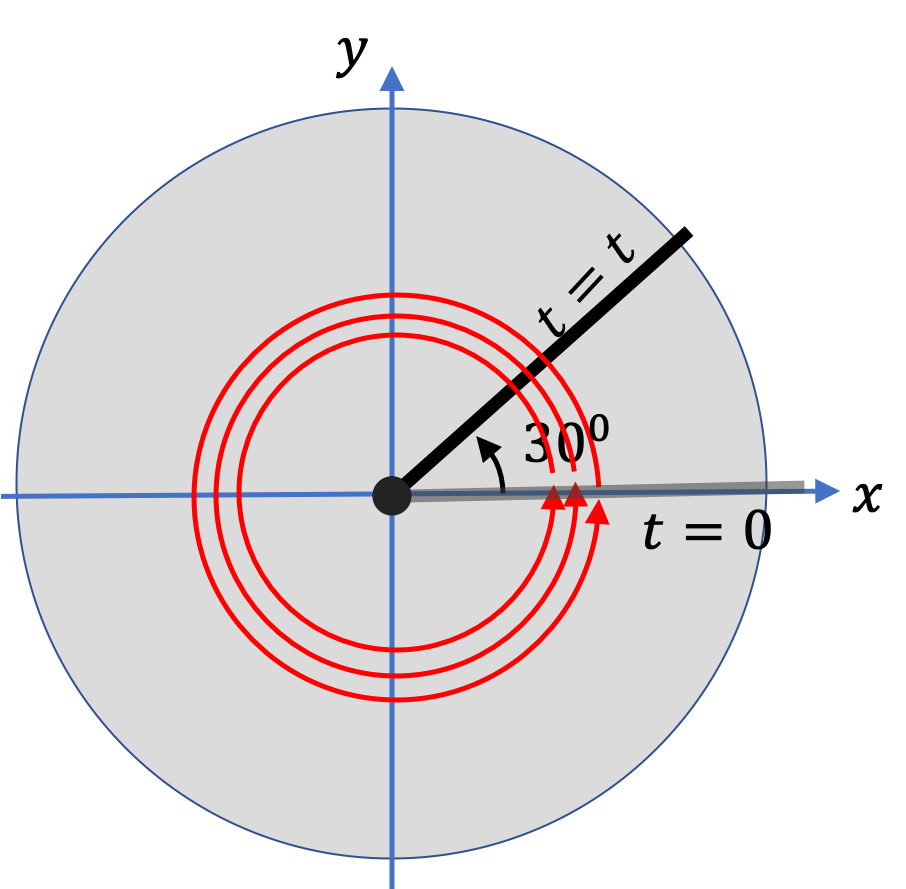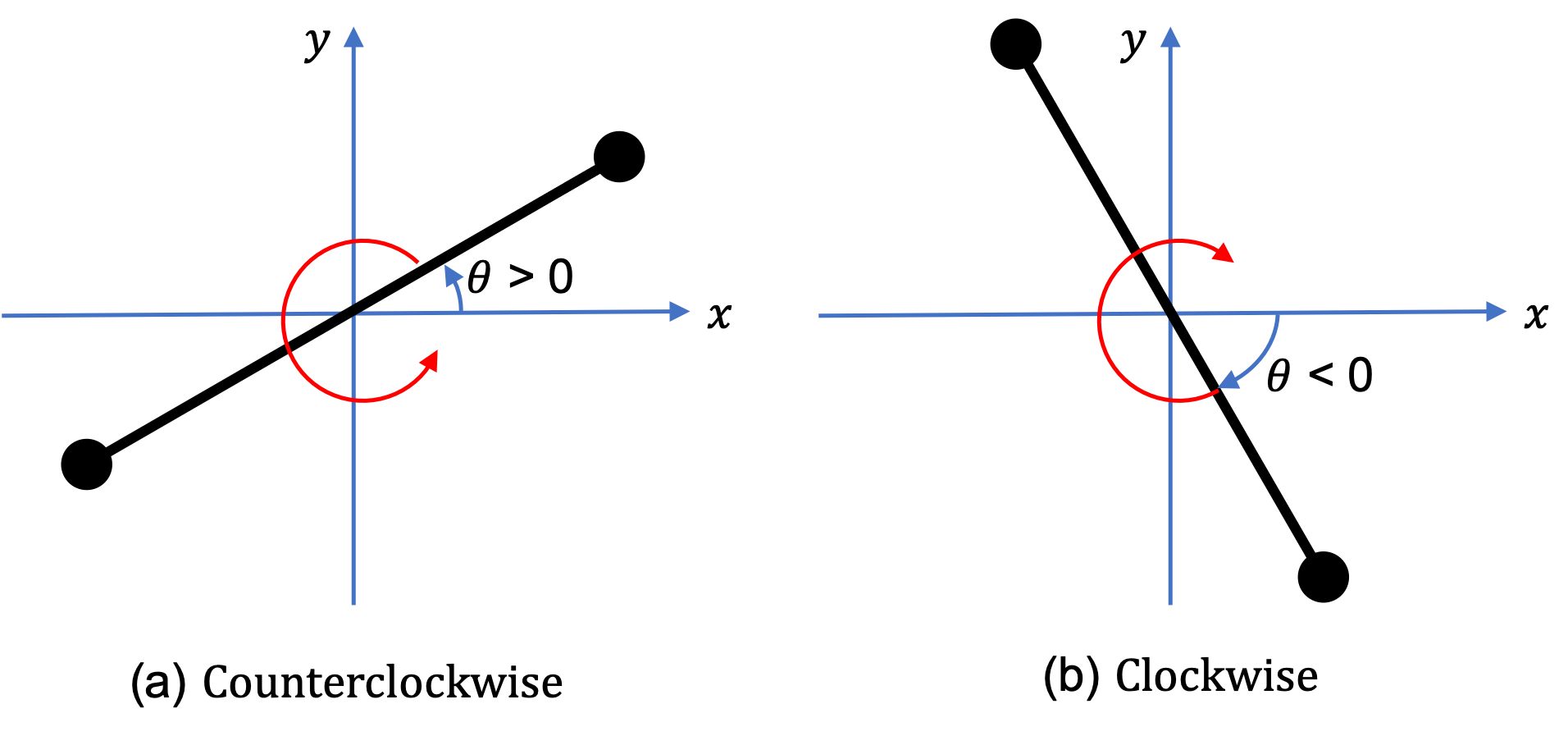## Section9.1Describing Rotation

The motion of changing orientation of an object is called rotation. When the body stays in one place while rotating, we call it a pure rotation. Following examples illustrate pure rotation where axis of rotation is fixed in time.

For instance, when a dancer performs a pirouette (see https://www.youtube.com/watch?v=fm-XZCi9skQ for a demonstration Dawid Trzensimiech of the Royal Ballet), the orientation of the dancer changes in-place - the dancer performs a pure rotation about an imaginary vertical line, which is the axis of rotation.

In a merry-go-round, the merry-go-round rotates about the center pole, which itself rotates. But, if you imagine a line through the very center, you will notice that everything is rotating about that line. The line is the axis of rotation.

Drill a hole through a block of wood and place a pin of smaller diameter than the hole. Now, when you give the block a sideways force, the block will rotate about the pin. The pin is the axis of rotation.

To quantify the amount of rotation, we imagine a line from the axis to any point in the rotating body. You will find that the line rotates with the body as illustrated in Figure 9.1.4. Rotation is quantified by an angle about the axis of rotation. In the figure, the disk has rotated by three full rotations and angle $30^\circ\text{.}$ We usually, write the angle in radians, which will make the rotation to be $\theta = 3\times 2\pi + \pi/6$ radians.Figure 9.1.4. Quantifying rotation by following the orientation of a marked line on the rotating body. The rotation angle here is 3 full rotations plus $30^\circ\text{,}$ which gives $\theta = 3\times 360 + 30 = 1110^\circ\text{.}$ We can also indicate this rotation as $3+\frac{1}{12}$ revolutions or as $3\times 2\pi + \pi/6$ radians.

### Subsection9.1.1Sense of Rotation

The direction of rotation is often specified by what is called the sense of the rotation. There are two senses of rotation:

1. Clockwise Rotation.
2. Counterclockwise Rotation.

When we look at a rotating body from the axis of rotation, the rotating object may appear to move clockwise or counterclockwise. If it appears to move clockwise, we say that the rotation is clockwise from that end. On the other hand, if the body appears to rotate counterclockwise, we have a counter-clockwise rotation. In the figure, the sense of rotation is counterclockwise.

The sense of rotation is not a very good way of telling direction of rotation since the answer depends upon which side of a rotating body you look from. For instance, if a rotating disk from above appears as counter-clockwise, then from below, it will be clockwise. Despite this ambiguity, the language of clockwise and counterclockwise has become so ingrained that it is impossible to get rid of it.

### Subsection9.1.2Angle of Rotation

The amount of rotation is quantified by the angle with which the body has rotated. A full rotation is called one revolution, $2\pi$ radians or $360$ degrees. The fraction of a full rotation are expressed as fractions of these quantites. Although, we will use degrees and revolutions as measures of rotation, we will use the radian for the angle in most of our calculations.

If angle of rotation exceeds one full roation, we keep adding the angles. Thus, if a rotation is one and half revolution, we have angle equal to $1.5$ revolutions, $2\pi + \pi = 3\pi\text{ rad}\text{,}$ or $360^{\circ} + 180^{\circ} = 540^{\circ}\text{.}$

We take the sense of rotation into account by including a sign for the value of the angle. If the sense of rotation is clockwise, we say that the angle of rotation is negative, and if the sense is counterclockwise, the angle is positive. These are illustrated in Figure 9.1.6

The sign convention is consistent with the right-handed $Oxyz$ coordinate system. Suppose the axis of rotation is along the $z$ axis, and we are looking on the rotating body from the positive $z$ axis direction. Let $\theta$ be the angle of rotation with respect to the positive $x$ axis. Then, you will notice that, for a clockwise rotation $\theta \lt 0\text{,}$ and for a counterclockwise rotation $\theta \gt 0\text{,}$ which is consistent with the sign convention.Figure 9.1.6. The angle of rotation is positive for a counterclockwise rotation and negative for a clockwise rotation. Note that the positive $z$ axis, not shown here, is pointed out of the page, which means we are looking at the rotating body from the direction of the positive $z$ axis, which is the axis of rotation in this figure.

A disk is rotating about an axle through its center. A line is marked on the disk from the axle to the edge of the disk to follow the rotation. When the disk rotates, the line rotates with it.

Choose a Cartesian coordinate system so that, at $t=0\text{,}$ the line on the disk is in the direction of the positive $x$ axis.

(a) When the disk is spinning counterclockwise, in $10 \text{ sec}$ the line went around four times and ended up at $90^{\circ}$ counterclockwise from the positive $x$ axis. What is the total angle rotated in this time? Express your answer in (i) degrees, (ii) radians, and (iii) revolutions.

(b) When the disk is spinning clockwise, in $10 \text{ sec}$ the line went around four times and ended up at $90^{\circ}$ counterclockwise from the positive $x$ axis. What is the total angle rotated in this time? Express your answer in (i) degrees, (ii) radians, and (iii) revolutions.

Hint

(a) Add up all the angles. (b) Be careful here, while the disk is rotating clockwise, the final orientation is given in counterclockwise angle.

(a) (i) $1530^{\circ}\text{,}$ (ii) $\dfrac{17}{2}\,\pi\text{ rad} \text{,}$ (iii) $4.25\text{,}$ (b) (i) $-1710^{\circ}\text{,}$ (ii) $-\dfrac{19}{2}\,\pi\text{ rad} \text{,}$ (iii) $-4.75\text{.}$

Solution 1 (a)

(a) (i) The angle of rotation is the cumulative angle. So, we have 4 full rotations and $90^{\circ}$ more, all given in counterclockwise.

\begin{equation*} \theta = 4\times 360^{\circ} + 90^{\circ} = 1530^{\circ}. \end{equation*}

(ii) Since $180^{\circ} = \pi\text{ rad}\text{,}$ this angle is same as

(iii) We can convert the angle in (i) to revolutions, by noting that one revolution has $360^{\circ}\text{.}$

\begin{equation*} \theta = 1530^{\circ} \times \dfrac{ 1\text{ rev}}{360^{\circ}} =4.25\text{ revs.} \end{equation*}
Solution 2 (b)

(a) (i) The angle of rotation is the cumulative angle. so, we need all angles in the same sense. We have 4 counterclockwise full rotations and then some. That additional angle is given in clockwise, which we need to be counterclockwise, which will be obtained by subtracting $360^{\circ}$ from the counterclockwise angle. counterclockwise.

\begin{equation*} \theta = - 4\times 360^{\circ} + \left( 90^{\circ} - 360^{\circ} \right) = -1710^{\circ}. \end{equation*}

(ii) Since $180^{\circ} = \pi\text{ rad}\text{,}$ this angle is same as

(iii) We can convert the angle in (i) to revolutions, by noting that one revolution has $360^{\circ}\text{.}$